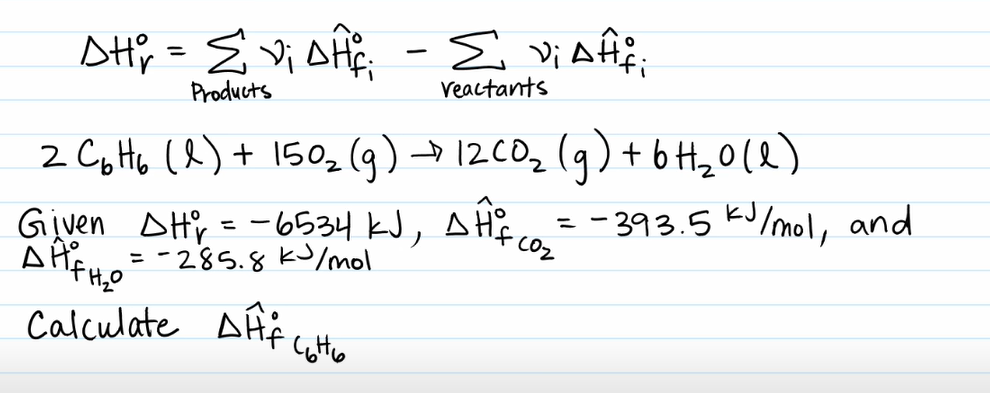LearnChemE

#### Heats of Reaction: Example Problems

Try to solve these problems before watching the solutions in the screencasts.

##### Example Problem 1##### Example Problem 3

Calculate the heat of reaction at 600 K when hydrazine (NH4) decomposes to form nitrogen and hydrogen. The constants for heat capacities, where CP is in J/(mol K) and T is in K, are in the table below.

$C_{P,i} = A_i + B_iT + C_iT^2 + D_iT^3$

 gas $$A_i$$ $$B_i$$ $$C_i$$ $$D_i$$ NH4 16.276 1.46E-01 -9.64E-05 2.51E-08 N2 28.883 -1.57E-03 8.08E-06 -2.87E-09 H2 29.088 -1.92E-03 4.00E-06 -8.7E-10
##### Example Problem 2

Determine the enthalpy change of this reaction using heats of formation and material balances. CO(g) + H2O(g) → CO2(g) + H2(g)

The reactants come in at 300°C and the products leave at 500°C.  The heats of formation for CO, H2O, and CO2 are -110.53 kJ/mol, -241.83 kJ/mol, and -393.5 kJ/mol, respectively.